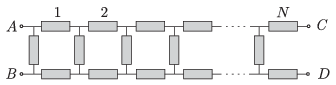Mathematical and Physical Journal
for High Schools
Issued by the MATFUND Foundation
 Already signed up? New to KöMaL?

#Problem P. 4681. (November 2014)

P. 4681. The chain of resistors made of $\displaystyle 3N$ resistors as shown in the figure can be closed in two different ways.$\displaystyle a)$ The terminals at points $\displaystyle A$ and $\displaystyle C$ are connected and also the terminals at point $\displaystyle B$ and $\displaystyle D$ are connected.

$\displaystyle b)$ The terminals at points $\displaystyle A$ and $\displaystyle D$ are connected and also the terminals at $\displaystyle B$ and $\displaystyle C$ are connected. (Möbius band.)

In which case will the equivalent resistance of the system between $\displaystyle A$ and $\displaystyle B$ be greater?

(6 pont)

Deadline expired on December 10, 2014.

### Statistics:

 13 students sent a solution. 6 points: Asztalos Bogdán, Balogh Menyhért, Berta Dénes, Blum Balázs, Csathó Botond, Fekete Panna, Forrai Botond, Holczer András, Kaposvári Péter, Sal Kristóf. 4 points: 2 students. 0 point: 1 student.

Problems in Physics of KöMaL, November 2014